## a circle with a circumference of 40.82 centimeters what is the area of the circle is 3.14 for pi​

Question

a circle with a circumference of 40.82 centimeters what is the area of the circle is 3.14 for pi​

in progress 0
6 months 2021-07-28T03:36:12+00:00 2 Answers 5 views 0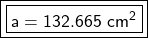Step-by-step explanation:

We are given the circumference of 40.82 centimeters. The formula for circumference is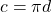The diameter is twice the radius, so we can also write this formula as: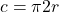Substitute 40.82 for the circumference and use 3.14 for pi.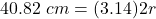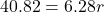6.82 and r are being multiplied. The inverse of multiplication is division. Divide both sides by 6.82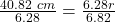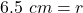2. Calculate the Area

Now we can calculate the area using this formula: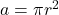The radius is 6.5 centimeters and we are using 3.14 for pi.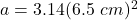Solve the exponent.

• (6.5 cm)²= 6.5 cm * 6.5 cm=42.45 cm²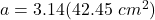Multiply.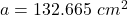The area is 132.665 square centimeters.

2. You need two formulas for this:

C = πd or C = 2πr ( d = 2r ) Where C is circumference and d is diameter, r is radius

A = πr² Where A is area, and r is radius

First you get the diameter and radius by substituting the value of pi and circumference. With that, you can calculate the Area of the circle with the second formula. square² the radius then multiply it to 3.14. Remember that radius is half of diameter.